# Diophantine approximation, metric theory of

(diff) ← Older revision | Latest revision (diff) | Newer revision → (diff)

The branch in number theory whose subject is the study of metric properties of numbers with special approximation properties (cf. Diophantine approximations; Metric theory of numbers). One of the first theorems of the theory was Khinchin's theorem ,  which, in its modern form , may be stated as follows. Let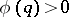be a monotone decreasing function, defined for integers. Then the inequalities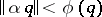have an infinite number of solutions in integersfor almost-all real numbersif the series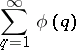(1)

diverges, and have only a finite number of solutions if this series converges (here and in what follows,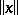is the distance fromto the nearest integer, i.e.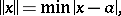where min is taken over all integers; the term "almost-all" refers to Lebesgue measure in the respective space). The theorem describes the accuracy of the approximation of almost-all real numbers by rational fractions. For example, for almost-allthere exists an infinite number of rational approximations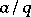satisfying the inequality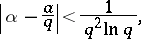whereas the inequality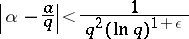has for anyan infinite number of solutions only for a set of numbersof measure zero.

The generalization of this theorem to simultaneous approximations  is as follows. The system of inequalities(2)

has a finite or infinite number of solutions for almost-all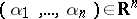depending on whether the series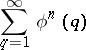(3)

converges or diverges.

More extensive generalizations refer to systems of inequalities in several integer variables .

A distinguishing feature of Khinchin's theorem and its many generalizations is the fact that the property of "convergence-divergence" of series of the types (1), (3) serves as a criterion of the corresponding order of the approximation applying to a set of numbers of measure zero or to almost-all numbers. It is a kind of "zero-one" law for the metric theory of Diophantine approximations. Another characteristic of these generalizations is the fact that the metric property of the numbers involved refers to a measure defined throughout the space containing the numbers which participate in the approximation, and that the measure of the space is defined as the product of the measures in the coordinate spaces. For instance, in the case of system (2) one speaks about an approximation of"independent" numbers and about the Lebesgue measure in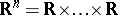(times). In this connection this part of the theory received the name of metric theory of Diophantine approximations of independent variables. It has been fairly thoroughly developed, but a number of unsolved problems still (1988) remain. One such problem concerns the conditions which must be imposed on a sequence of measurable sets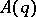,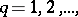in the interval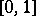for the convergence or divergence of the series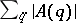to correspond to the condition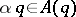to be satisfied a finite or infinite number of times for almost-all. A similar problem arises for the system of numbers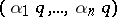.

The metric theory of Diophantine approximations of dependent variables, which is of a later date, immediately gave rise to several fundamental and characteristic problems . The first one originated in the theory of transcendental numbers (Mahler's conjecture) and concerned simultaneous rational approximations to a system of numbers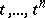for almost-allfor any fixed natural number. A recent result obtained on this subject runs as follows. Letbe a monotone decreasing function for which the series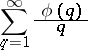converges. Then the system of inequalities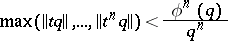has only a finite number of solutions in integersfor almost-all.

This theorem confirms that it is possible to approximate by rational numbers almost-all the points of a curve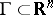. Considerations of more general manifolds inwill yield similar results.

If almost-all (in the sense of the measure on) points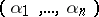of the manifoldare such that the system (2) with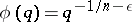has a finite number of solutions in integers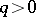for any,is said to be extremal, i.e. almost-all points permit only the worst simultaneous approximation by rational numbers. Schmidt's theorem says that ifis a curve in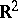with non-zero curvature at almost-all its points, it is extremal .

The method of trigonometric sums (cf. Trigonometric sums, method of; see also Vinogradov method) makes it possible to detect extremality of very general manifoldsin, under the condition that the topological dimension. If, on the other hand,, the extremal manifold cannot be quite general, and its structure should be fairly definite .

How to Cite This Entry:
Diophantine approximation, metric theory of. Encyclopedia of Mathematics. URL: http://encyclopediaofmath.org/index.php?title=Diophantine_approximation,_metric_theory_of&oldid=19083
This article was adapted from an original article by V.G. Sprindzhuk (originator), which appeared in Encyclopedia of Mathematics - ISBN 1402006098. See original article